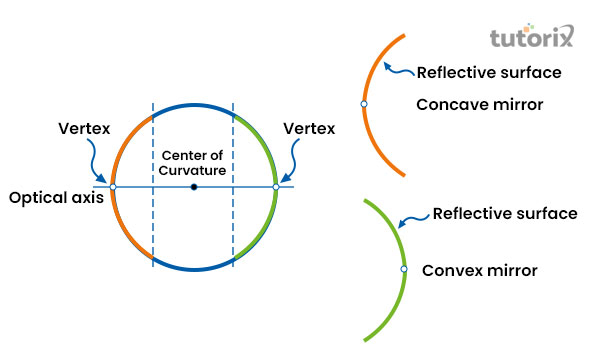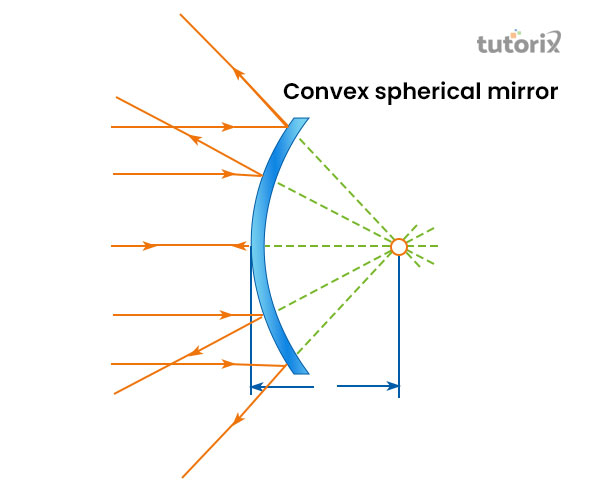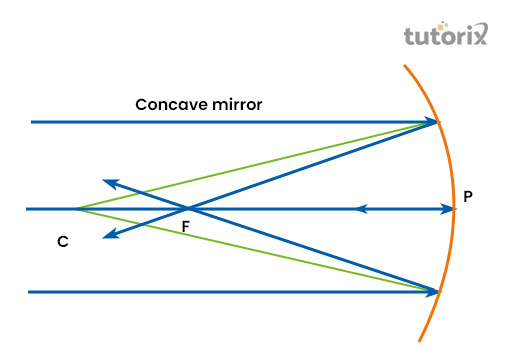# Spherical Mirror Formula

## Introduction

Spherical mirrors are commonly used in daily human lives starting from making automobile headlights to torch. The mirror involves two spherical surfaces, convex and concave in order to form a true shepherd. A spherical mirror is commonly made from the cut-outs of spherical surfaces. This type of mirror has a major function in terms of creating images by following a certain formula of reflection and magnification in real-time.

## What is Spherical Mirror

A spherical mirror can be defined as a mirror that incorporates a hollow sphere while creating its reflecting surface while creating reflections and images. A spherical mirror involves key factors like curvature centre, principal axis, pole, principal focus, curvature radius, focus, and aperture (Phys, 2022).

Spherical mirrors generally consist of both convex and concave mirrors to reflect images based on the position of the light.Figure1: Spherical mirror

Characteristically, mirrors are symmetrical with a principal axis or optical axis that reflects the optical elements and a vertex in the centre of curvature for the mirror.

In the case of the spherical mirrors, both the inward and the outward surfaces act as reflective surfaces based on the pathway of light (Cnx, 2022). In a spherical lens, the optical axis and the vertex conjugates in the centre of curvature to make things optical for human eyes in real-time.

Types of Spherical Mirrors

Based on the way of reflecting images, the spherical mirrors can be categorised into two different parts, the convex mirror and the concave mirror.

## Convex Mirror

Convex mirror is a spherical mirror where the reflective surface of the mirror commonly bulges out towards the source of light. In the case of this mirror, the reflection is directed to the outward side from the focal point. Within a convex mirror the centre of curvature and the focal point cannot be reached at the same time, so it forms a virtual image as a replica of the reflection (Poplavskiy et al. 2020). As a result, the process of image formation cannot be projected inside the mirror.Figure 2: Convex mirror

The formed image within a convex mirror becomes bigger when the object comes close to the mirror. For example, if a numbered slate is placed at a longer distance from a human eye, the image of the numbered slate will look smaller at first. But as soon as the numbered slate is placed close to the human eye, it will look bigger to the eye as the rule of the convex mirror.

## Concave Mirror

A concave mirror involves a reflective curve in the inward direction, away from the source flight. Characteristically, the concave mirrors reflect light in the inward direction to the focal point of the mirror (Karpov & Horak, 2021). The images formed by the concave mirrors generally show different kinds of images when objects and mirrors are placed in different directions at a time.Figure 3: Concave mirror

In a concave mirror light generally converges at a certain point whenever it strikes a surface and reflects back from it. The convergence within the mirror earned it the name of converging mirror. Concave mirrors mostly create a magnified, virtual and an erect image when an object is placed at a very close distance from the mirror. On the other hand, when the distance between the object and the mirror is increased, it reduces the image and forms an inverted image in the process (Peda, 2022). This type of mirror is capable of forming both real as well as virtual images under different circumstances.

## The formula for Spherical Mirror

The formula for spherical mirror allows explaining the common relationship between an object distance, focal length of a spherical mirror and an image distance. In the formula, the object and the pole are denoted by u and distance between the pole and the image is denoted by v. The focal length of the spherical mirror is denoted by f in this formula. So, the formula here stands as 1/v+1/u=1/f

The present formula explains that the object distance (u), image distance (v) and the focal length (f) of a spherical mirror is interrelated. Most importantly, this formula applies to almost every spherical mirror under the different positions of the object placed in front of the mirror.

## Conclusion

Spherical mirrors are generally the cut-outs of mirrors that have curved surfaces painted on both the inward and outward sides of the mirror. Spherical mirrors are segmented between convex lens and concave lens. In a convex mirror light generally flows in the outward direction and makes the image smaller than the actual object from the distance. The images formed in the concave mirrors can be large or small depending on the distance between the mirror and the object. The formula for spherical mirror depends on three factors, the object distance, image distance and focal length where it stands as 1/v + 1/u = 1/f.

## FAQs

Q1. What the diameter of a spherical mirror is called?

The diameter of a spherical mirror is called the aperture. It acts as the reflecting surface of the mirror when light falls on it.

Q2. When a plane mirror can be called a spherical mirror?

Due to the infinite radius of curvature within a plane mirror, it can sometimes be termed a spherical mirror. For example, the curvature radius of an automobile looking glass can be recognized as a spherical mirror.

Q3. Which rules do the spherical mirrors mostly follow?

The spherical mirrors mostly follow the rule of focus when a ray of light falls parallel to the principal axis of the mirror. In addition, it also follows the rule of curvature when a ray of light goes directly towards the centre of the curvature when reflecting back in the same path it came.

## References

### Journals

Karpov, D. V., & Horak, P. (2021). Cavities with Non-Spherical Mirrors for Enhanced Quantum Emitter-Cavity Photon Interaction. arXiv preprint arXiv:2110.05981. Retrieved from: https://arxiv.org

Poplavskiy, M. V., Matsko, A. B., Yamamoto, H., & Vyatchanin, S. P. (2020). Diffraction losses of a Fabry-Perot cavity with nonidentical non-spherical mirrors. Journal of Optics, 22(11), 115603. Retrieved from: https://arxiv.org

## Websites

Cnx, (2022). Definition of spherical mirrors. Retrieved from: https://cnx.org [Retrieved on 15th June 2022]

Peda, (2022). The formula of spherical mirrors. Retrieved from: https://peda.net [Retrieved on 15th June 2022]

Phys, (2022). Spherical mirrors. Retrieved from: https://phys.libretexts.org [Retrieved on 15th June 2022]